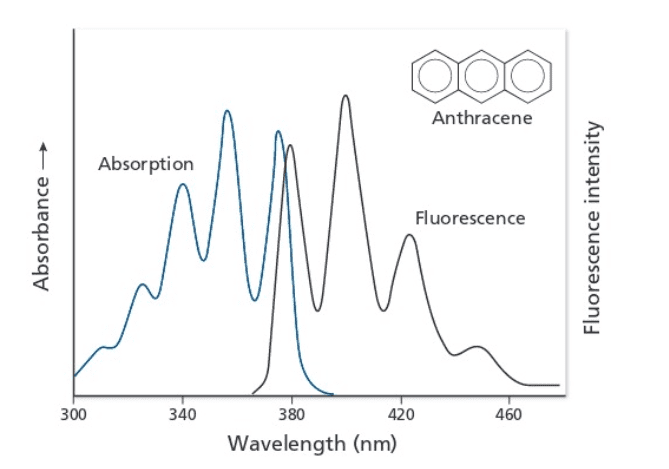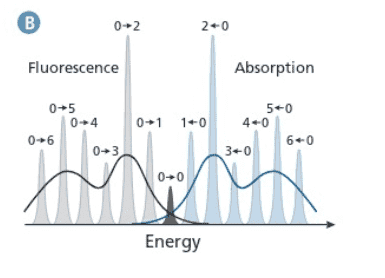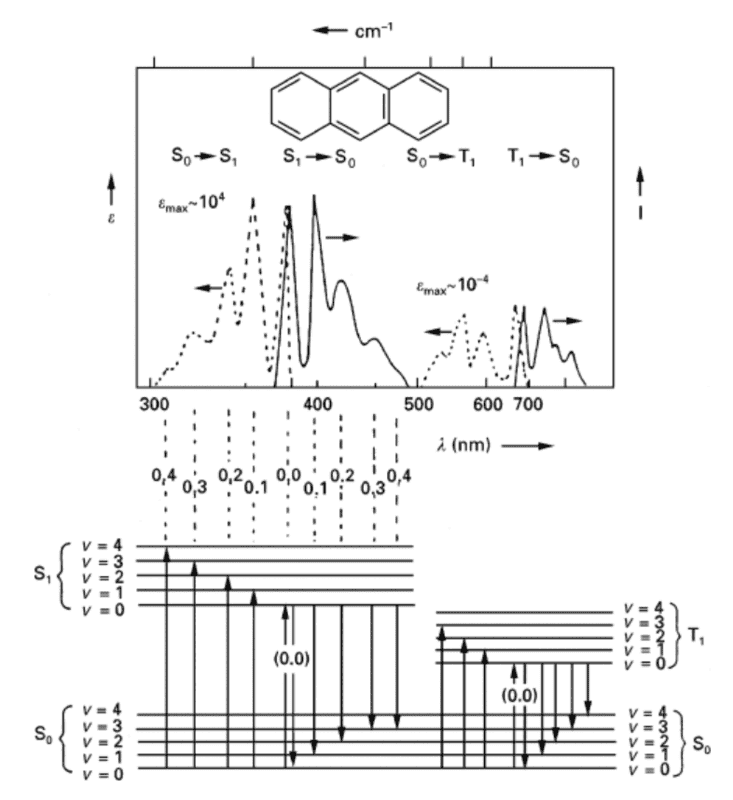# Determine energy state difference using absorption-emission spectra

JoJoQuinoa
Hello,

I was wondering if someone could help clarifying this question.

The question asks to estimate the energy state difference between the vibrational ground state of S0,v=0 and the first excited vibrational ground state S0,v=1 of the spectra below.

The given solution: S1,v=1 -> S0,v=1 at $\lambda$ = 400 nm and S1,v=2 -> S0,v=1 at $\lambda$ = 380 nm.

There are two things I'm confused about the solution:
1) From Figure B, I would assume that the first Fluorescence peak at 380 nm in Figure A corresponds to S1,v=0 -> S0,v=1 and the second peak at 400 nm corresponds to S1,v=0 -> S0,v=2. Larger transition results in higher emission energy or smaller wavelength.

2) Why is S0,v=1 being used as the final state for both peaks?
The S1,v=1 -> S0,v=1 would occur as S1,v=1 ->S1,v=0 ->S0,v=1 and
S1,v=2 ->S1,v=0 ->S0,v=1.
Since S1,v=2 or 1 ->S1,v=0 is internal conversion, wouldn't S1,v=0 ->S0,v=1 for the two transitions give off the same energy?Gold Member
I don't think the solution matches what the question asks for. Could you write the fully replicated question and the solution? Perhaps, a screenshot/photo of the question and solution?

For example, the question asks for the energy state difference, which means that the solution should be in the units of eV or cm-1 (or Hartree). It doesn't make any sense that the solution is in wavelength (nm) because wavelength is inversely proportional to energy and is not linear. Thus, you can't just add/subtract wavelength differences (unless you know what you are doing).

Also,
1) No, the fluorescence peak at 380 is not S1,v=0 → S0,v=1, and the second peak at 400 nm is not S1,v=0 → S0,v=2. What is your reasoning that you are not seeing any v=0→0 transition?

•JoJoQuinoa
JoJoQuinoa
Hi Hayao,

The question was: Use the anthracene absorption and emission spectrum in Figure 8.5 (Figure 1 in this post) to construct a combined Jablonski diagram/absorption/emission spectrum sketch. Label all states and calculate transition energies in cm-1.

The solution provided by the Professor and the one I found on Google were similar and as provided in the original post: S1,v=1 -> S0,v=1 at λ = 400 nm and S1,v=2 -> S0,v=1 at λ = 380 nm. I believe both were mislabeled. I found the figure below from ScienceDirect and I think it is correct.

"No, the fluorescence peak at 380 is not S1,v=0 → S0,v=1, and the second peak at 400 nm is not S1,v=0 → S0,v=2. What is your reasoning that you are not seeing any v=0→0 transition?"

Initially, I labeled the first peak as v=0->0 (overlapping peaks in Absorption and Emission spectrum are v=0->0 transition) and second peak to be v=0->1. I got confused by the solution and modified my answer.•